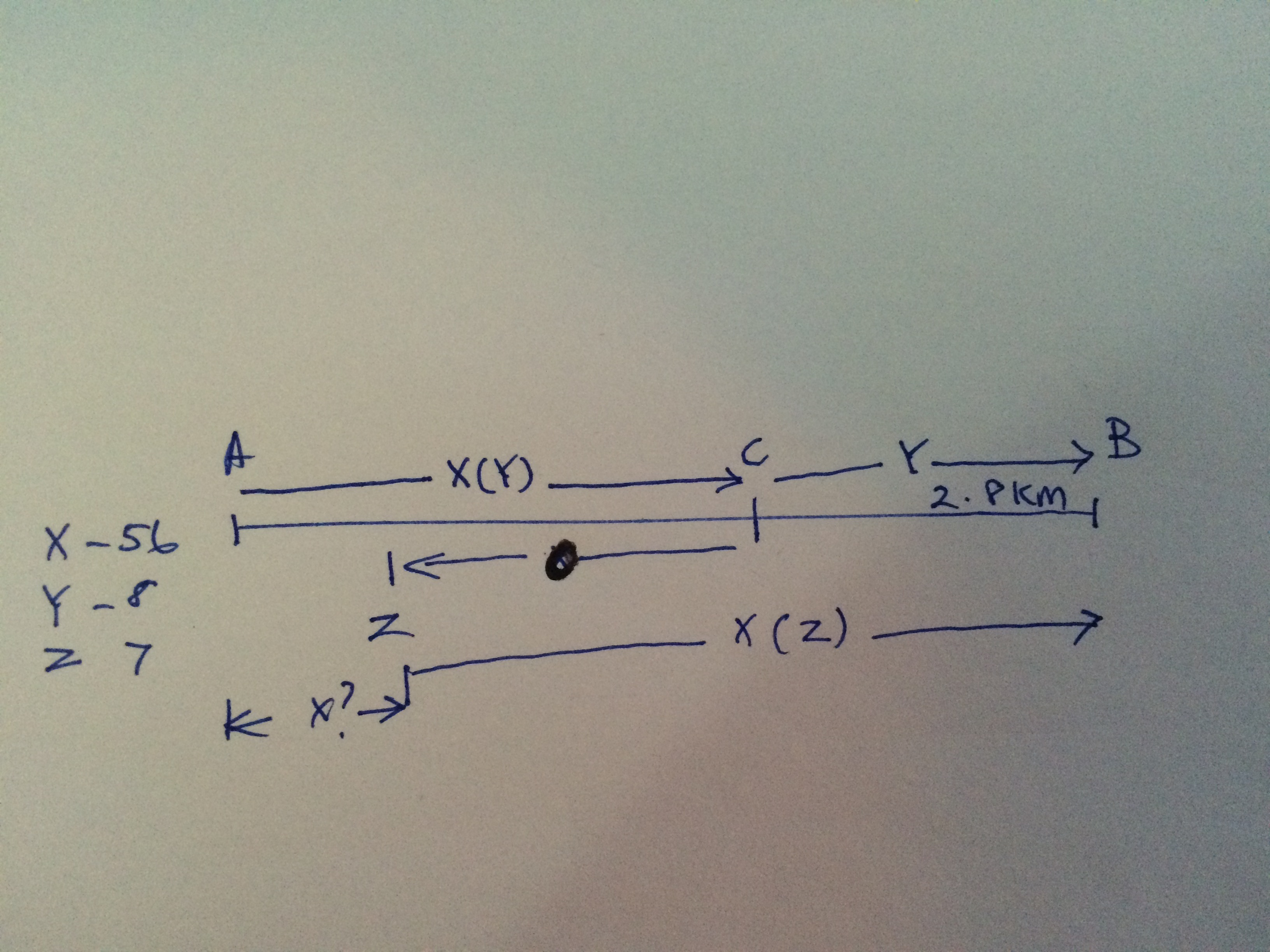# Solving wordy problems step-by-stepThree persons X,Y,Z travel from point A to point B . They leave point A at the same time and follow the same route. X rides a motorcycle at a speed of 56 km/h, Y walks at a speed of 8 km/h and Z walks at a speed of 7km/h. At the beginning, X gives a lift to Y at A to travel a part of the journey and then comes back to pick up Z . They all arrive at point B at the same time. Given that Y walks 2.8 km. The distance which Z walked is x km. Find 10x. Question by Peng Ying Tan, a Brilliant user.

At first glance, this word problem seems gnarly, punctuated with many variables and unknowns. So, the best way to approach is to break it down into parts and perhaps, sketching a diagram might be a useful guide as some of us are more visually inclined than others.

Drawing a diagram helps with understanding the question. Since we are given the speed of each person, the other two major factors are distance and time. The question ask us to solve for x, which is the distance travelled by person Z on foot In order to know that, we would have to find the time elapsed for Z , and with his walking speed of 7 km/h, we can compute x.

The biggest hint , or common factor, in this word problem is all persons arrived at point B at the same time. In addition, we are told that Y walked for 2.8 km. Hence, the time it takes for Y to walk from C to B after getting dropped off by X ( see picture) is 2.8km/8km/h= 0.35 hr. ( km unit cancels off, as Distance/Speed=Time)

By this time, X would have travelled back to meet Z in some distance, lets call it O, picked up Z, and reached B. ( Remember we are told that all of them reached B at the same time!) So, in the span of 0.35 hours, how many miles did X travelled? X would have travelled back by a distance of O, pick up Z, travelled another distance of O, and plus the 2.8 km to reach B. Algebraically, (2*O+ 2.8)km / 56km/h=0.35 hrs. Solve for O.

O= 8.4 km=distance travelled by X after dropping Y off.

The a-ha moment: All started at the same time at A. When X travelled from A to C, dropped Y off, travelled back 8.4km back to Z, how much has Z travelled ( x)? (Note: By the time X got back to Z, X would have travelled x+16.8)

Since they are meeting for the first time , we can also claim that X and Z's time have elapsed equally, or t. In other words, elapsed time for Z= x km/ 7km/h= (8.4+8.4+x)km/56= elapsed time for X. Solve for x.

x = 2.4 km. 10x=24Note by Venture Hi
6 years, 4 months ago

This discussion board is a place to discuss our Daily Challenges and the math and science related to those challenges. Explanations are more than just a solution — they should explain the steps and thinking strategies that you used to obtain the solution. Comments should further the discussion of math and science.

When posting on Brilliant:

• Use the emojis to react to an explanation, whether you're congratulating a job well done , or just really confused .
• Ask specific questions about the challenge or the steps in somebody's explanation. Well-posed questions can add a lot to the discussion, but posting "I don't understand!" doesn't help anyone.
• Try to contribute something new to the discussion, whether it is an extension, generalization or other idea related to the challenge.

MarkdownAppears as
*italics* or _italics_ italics
**bold** or __bold__ bold
- bulleted- list
• bulleted
• list
1. numbered2. list
1. numbered
2. list
Note: you must add a full line of space before and after lists for them to show up correctly
paragraph 1paragraph 2

paragraph 1

paragraph 2

[example link](https://brilliant.org)example link
> This is a quote
This is a quote
    # I indented these lines
# 4 spaces, and now they show
# up as a code block.

print "hello world"
# I indented these lines
# 4 spaces, and now they show
# up as a code block.

print "hello world"
MathAppears as
Remember to wrap math in $$ ... $$ or $ ... $ to ensure proper formatting.
2 \times 3 $2 \times 3$
2^{34} $2^{34}$
a_{i-1} $a_{i-1}$
\frac{2}{3} $\frac{2}{3}$
\sqrt{2} $\sqrt{2}$
\sum_{i=1}^3 $\sum_{i=1}^3$
\sin \theta $\sin \theta$
\boxed{123} $\boxed{123}$